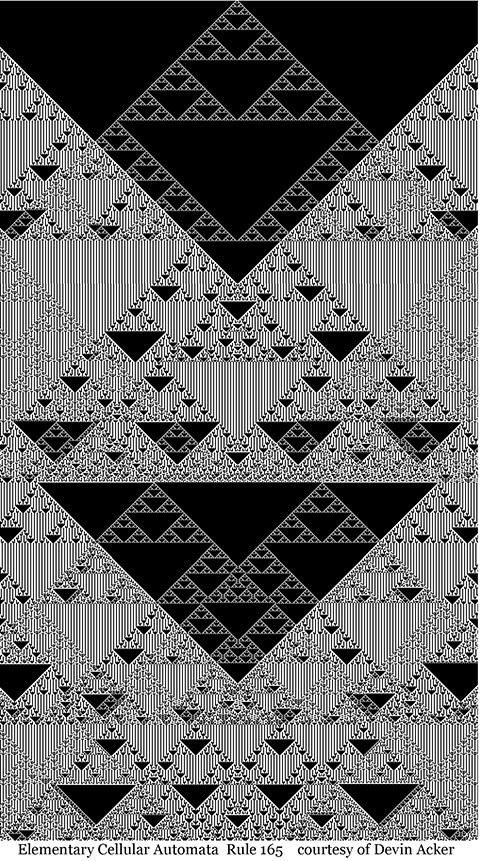# A METHODOLOGY OF INTUITIONFour Operations and Emergence

Mathematician Stephen Wolfram developed the cellular automata programs that demonstrate the fundamental premise that simple rules can generate complex patterns and behaviors. These simple rules designate pixels as being either on or off. The resulting graphs exhibit complex emergent patterns.

Information based transformations can be imaged through a variety of programs. Here, elementary cellular automata is used, which studies complexity through simple operations on binary elements over many iterations. A rich variety of patterns is generated with a total of 256 possible combinations.

“Elementary cellular automata have two possible values for each cell (0 or 1), and rules that depend only on nearest neighbor values. As a result, the evolution of an elementary cellular automaton can completely be described by a table specifying the state a given cell will have in the next generation based on the value of the cell to its left, the value the cell itself, and the value of the cell to its right.”
ElementaryCellularAutomaton

# 000      010       101       111

The four operations lined up in sequence represent rule 165 (out of 256 possible elementary cellular automata permutations). The results when the program is run appears below.  Only through many iterations of recorded history of the system is the complexity revealed.  It is an interesting idea to consider similar complexity happening as a result of  ontological operations.Below is rule 165.